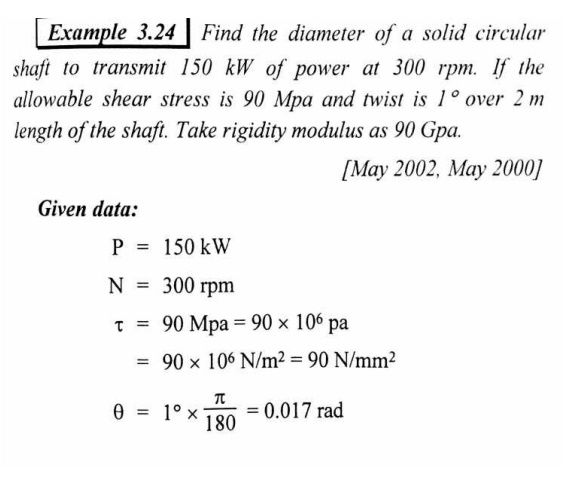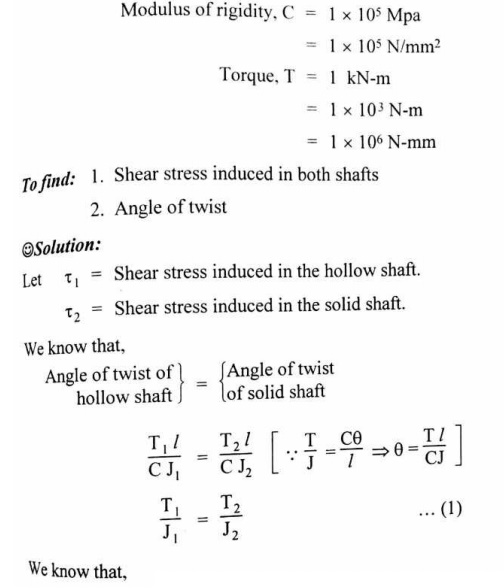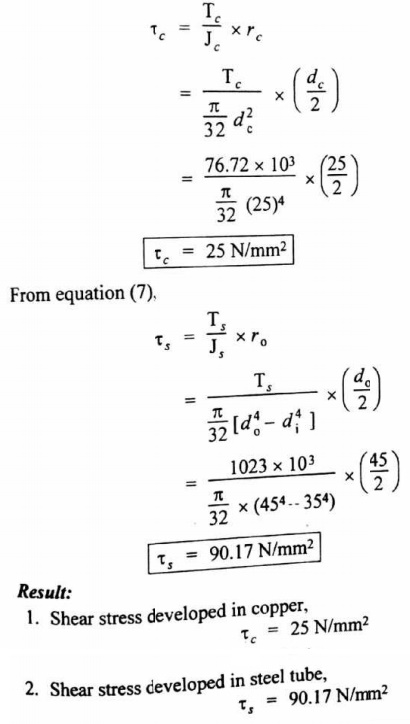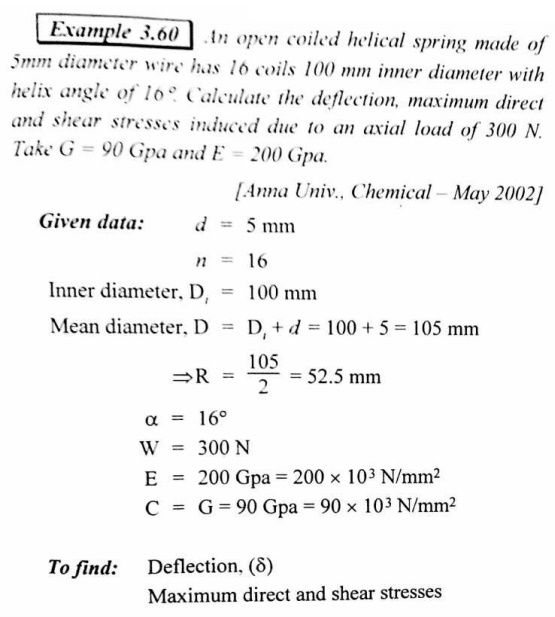Home | | Mechanics of Solids | Important Question, Answer And Solved Problems: Civil - Mechanics Of Solids - Torsion

# Important Question, Answer And Solved Problems: Civil - Mechanics Of Solids - Torsion

Civil - Mechanics Of Solids - Torsion

TORSION

1.  Define the term 'torque'.

The product of turning force, and the distance between the point of application of the force, and the axis of the shaft is known as torque..

2.     What are the assumptions made in theo ry of torsion.

(i)          The material of the shaft is uniform throughout.

(ii)       The twist along the shaft is uniform.

(iii)     The shaft is of uniform circular se ction throughout.

(iv)      Cross-Sections of the shaft, whic h are plane before twist, remain plane after t wist.

3.     Define the term polar modulus.

Polar modulus is defined as the ratio of the polar moment of inertia to the radius of the shaft.

Zp = J/R

4.  Define Torsional Rigidity.

We know the torsion equation

T/J = C?/l

? = Tl/CJ

Since C, l and J are constant for a given shaft, ? (angle of twist) is directly proportional to T (torque). The term CJ is known as torsional rigidity.

5.  Define the term 'equivalent bending moment'.

Equivalent bending moment (Me) may be defined as the bending moment which will produce the same direct stress as produced by the bending moment and the torque acting separately.

6.  Define the term 'equivalent twisting moment'

The equivalent twisting moment (Te) may be defined as the torque which will produce the same maximum shear stress as produced by the bending moment and the torque separately.

7.  Why hollow circular shafts are preferred when compared to solid circular shaft?

i). The torque transmitted by the hollow shaft is grater than the solid soft.

ii). For same material, length and given torque, the weight of the hollow shaft will be less compared to solid shaft.

8.  What is meant by spring?

Spring is a device which is used to absorb energy by very large change in its form without permanent deformation, and then release the same when required.

9.  What is meant by stiffness?

The stiffness of the spring is defined as the load required to produce unit deflection.

10. What are the different types of springs?

i). Torsion spring ii). Bending spring

11. What is torsion spring?

A torsion spring is the one which is subjected to a twisting moment and the resilience is mainly due to torsion.

12. What is bending spring?

A bending spring is one which is subjected to bending only and resilience is mainly due to bending.

13.  What are the stresses are induced in the spring?

i). Direct stress ii). Torsional stress iii). Bending stress

14. Explain the springs in parallel.

When two springs are joined in such a manner that they have common deflection they are said to be connected in parallel.

15. Explain the springs in series.

In many situations, the combination of two or more springs either may be connected in series or parallel are required.

16. What are the applications of closed coiled helical spring?

The closed coiled helical springs are used in Railway wagons, cycle seating, pistols, brakes etc.

17. Differentiate between close coiled and open coiled helical springs.

Close-coiled spring

a)     Adjacent coils are very close to each other

b)    Only tensile load can carry

c)     Helix angle is negligible

Open- coiled spring

a)     Large gap between adjacent coils

b)    Tensile and compressive loads can carry.

c)     Helix angle considerable

18. What is meant by stiffness of a spring and write an expression for it.

Stiffness (K) of a spring is a measure of its capacity and is defined as the load required producing unit deflection. K = P/d Where, P - Load, d - Deflection

19. What is buffer spring?

Buffer spring is mostly used in Railway wagons. The shock between two colliding bodies may be softened or cushioned by means of buffers.

20. Write an expression for the angle of twist for a hollow circular shaft with external diameter D, internal diameter d, length l and rigidity modulus G.

Angle of twist q = Tl/GJ Where, T- Torque

J-Polar moment of inertia J = p[D4 - d 4]/32

21.            Why hollow circular shaft are preferred when compared to solid circular shafts?

a) The torque transmitted by the hollow shaft is grater than the solid shaft.

b) For same material, length and given torque, the weight of the hollow shaft will be less Compared to solid shaft

22. Write down the equation for shear strain energy of closed coiled spring.

 Shear strain energy U= [f2 s/4C] x volume of the spring Where fs - Shear stress, C- Modulus of rigidity

23.            What kind of stress introduced when an axial load acts on an open coiled spring?

Bending stress and Shear stress

24.            What is meant by spring constant or spring index?

Spring constant is the ratio mean diameter of the spring to the diameter of the wire.

25.            What kind of stress induced when an axial load acts on a close coiled spring.

Shear stress.

26.            Explain leaf springs?

Leaf springs are called as laminate springs are commonly used in carriages such as Cars, Lorries and Railway wagons.

27. What are the applications of leaf spring?

Leaf springs are commonly used in carriages such as Cars, Lorries and Railway wagons shocks of vehicles given unpleasant felling to the passengers and hence springs are used to absorb such shocks.

28. What types of stresses are caused in a beam subjected to a constant shear force?

Vertical and horizontal shear stress.Study Material, Lecturing Notes, Assignment, Reference, Wiki description explanation, brief detail
Civil : Mechanics Of Solids : Torsion : Important Question, Answer And Solved Problems: Civil - Mechanics Of Solids - Torsion |

Related Topics